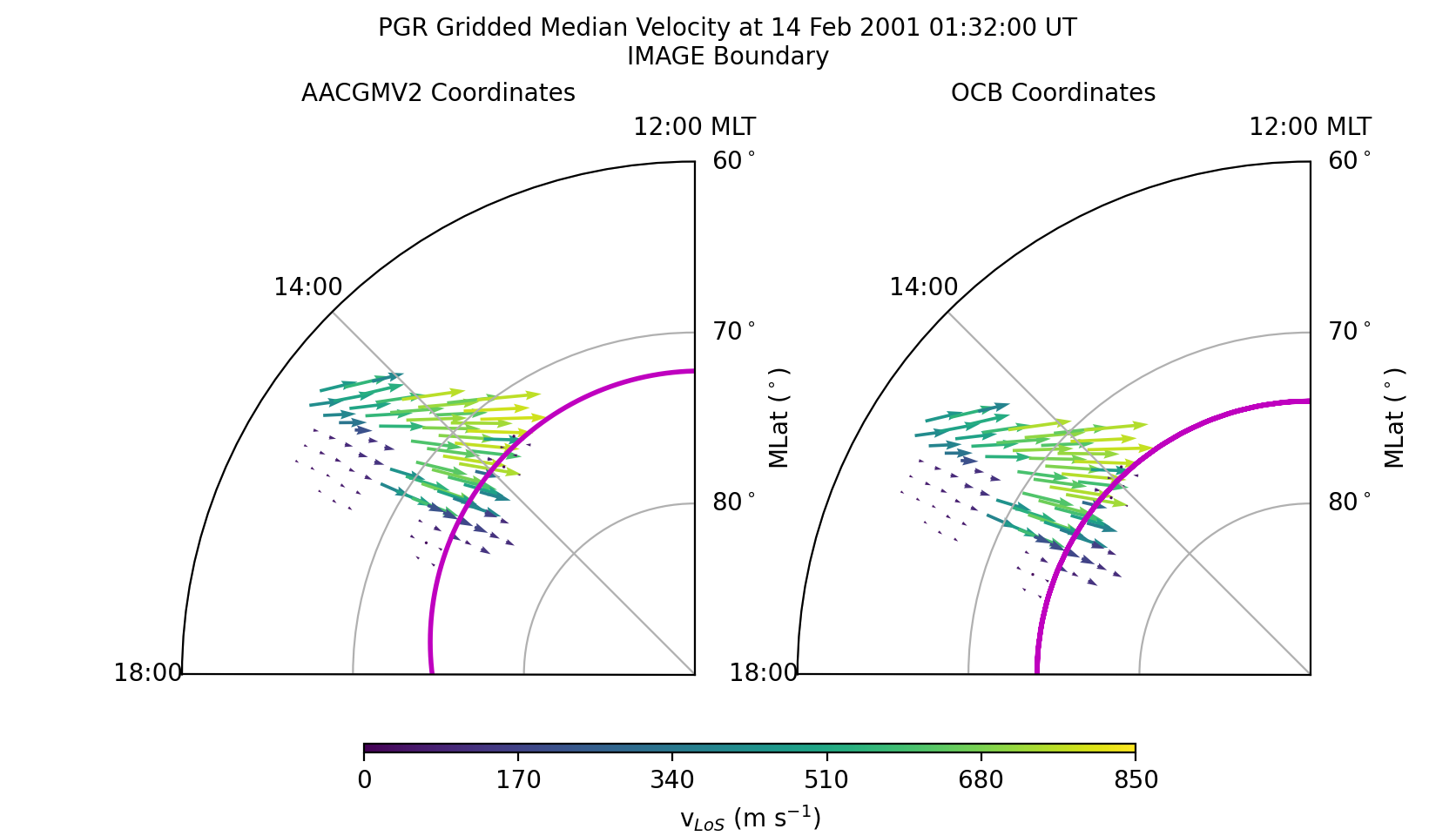# Grid and scale vector data¶

Many space science observations, such as ion drifts, are vectors. The ocbpy.ocb_scaling.VectorData class ensures that the vector location, direction, and magnitude are gridded and scaled appropriately.

The example presented here uses SuperDARN data. The example file, 20010214.0100.00.pgr.grd may be obtained by fitting and then gridding the rawacf file, available from any of the SuperDARN mirrors. FitACF v3.0 was used to create this file. See the Radar Software Toolkit for more information.

The SuperDARN data may be read in python using pydarn. To load this file (or any other grid file), use the following commands.

```import datetime as dt
import numpy as np
import matplotlib as mpl
import matplotlib.pyplot as plt

import aacgmv2
import ocbpy
import pydarn

filename = '20010214.0100.00.pgr.grd'
print(len(grd_data))

13
```

If you used the same file, there will be 13 grid records. Next, load the OCBs for the northern hemisphere (PGR is a Canadian radar) and the period of time available within this file.

```stime = dt.datetime(grd_data['start.year'], grd_data['start.month'],
grd_data['start.day'], grd_data['start.hour'],
grd_data['start.minute'],
int(np.floor(grd_data['start.second'])))
etime = dt.datetime(grd_data[-1]['start.year'], grd_data[-1]['start.month'],
grd_data[-1]['start.day'], grd_data[-1]['start.hour'],
grd_data[-1]['start.minute'],
int(np.floor(grd_data[-1]['start.second'])))
ocb = ocbpy.ocboundary.OCBoundary(stime=stime, etime=etime)
print(ocb)

Open-Closed Boundary file: ~/ocbpy/ocbpy/boundaries/si13_north_circle.ocb
Source instrument: IMAGE
Open-Closed Boundary reference latitude: 74.0 degrees

12 records from 2001-02-14 01:33:24 to 2001-02-14 01:55:54

YYYY-MM-DD HH:MM:SS Phi_Centre R_Centre R
------------------------------------------
2001-02-14 01:33:24 162.58 1.41 16.16
2001-02-14 01:35:26 176.90 1.77 16.16
2001-02-14 01:53:51 311.82 2.51 14.44
2001-02-14 01:55:54 193.16 2.67 15.63

Uses scaling function(s):
circular(**{})
```

To convert this vector into OCB coordinates, we first need to pair the grid record to an approriate boundary. In this instance, the first record in each case is appropriate.

```ocb.get_next_good_ocb_ind()
print(ocb.dtime[ocb.rec_ind]-stime)

0:01:24
```

If you are using a different file, you can use ocbpy.match_data_ocb to find an appropriate pairing, as illustrated in previous examples.

Now that the data are paired, we can initialize an ocbpy.ocb_scaling.VectorData object. To do this, however, we need the SuperDARN LoS velocity data in North-East-Vertical coordinates. SuperDARN grid files determine the median magnitude and direction of Line-of-Sight (LoS) Doppler velocities. For each period of time, the velocity is expressed in terms of a median vector magnitude and an angle off of magnetic north. To convert between the two, use the following routine.

```def kvect_to_ne(kvect, vmag):
return drifts_e, drifts_n

# Calculate the drift components
drifts_e, drifts_n = kvect_to_ne(grd_data['vector.kvect'],
grd_data['vector.vel.median'])

# Create an array of the data indices
dat_ind = np.arange(0, len(grd_data['vector.kvect']))

# Calculate the magnetic local time from the magnetic longitude
mlt = aacgmv2.convert_mlt(grd_data['vector.mlon'], stime)

# Initialize the vector data object
pgr_vect = ocbpy.ocb_scaling.VectorData(dat_ind, ocb.rec_ind,
grd_data['vector.mlat'], mlt,
aacgm_n=drifts_n, aacgm_e=drifts_e,
aacgm_mag=grd_data['vector.vel.median'],
dat_name='LoS Velocity',
dat_units='m s\$^{-1}\$',
scale_func=ocbpy.ocb_scaling.normal_curl_evar)

# Calculate the OCB coordinates of the vector data
pgr_vect.set_ocb(ocb)
```

Because there are 110 vectors at this time and location, printing `pgr_vect` will create a long string! Vector data does not require array input, but does allow it to reduce the time needed for calculating data observed at the same time. A better way to visualise the array of vector velocity data is to plot it. The following code will create a figure that shows the AACGMV2 velocities on the left and the OCB velocities on the right. Because data from only one radar is plotted, only a fraction of the polar region is plotted.

```# Initialize the figure and axes
fig = plt.figure(figsize([8.36, 4.8]))

# Format the axes
xticks = np.linspace(0, 2.0*np.pi, 9)
for aa in [axa, axo]:
aa.set_theta_zero_location('S')
aa.xaxis.set_ticks(xticks)
aa.xaxis.set_ticklabels(["{:02d}:00{:s}".format(int(tt), ' MLT'
if tt == 12.0 else '')
aa.set_rlim(0, 30)
aa.set_rticks([10, 20, 30])
aa.yaxis.set_ticklabels(["80\$^\circ\$", "70\$^\circ\$", "60\$^\circ\$"])
aa.set_thetamin(180)
aa.set_thetamax(270)
aa.yaxis.set_label_position('right')

fig.suptitle('PGR Gridded Median Velocity at {:} UT\n{:s} Boundary'.format(stime.strftime('%d %b %Y %H:%M:%S'), ocb.instrument.upper()), fontsize='medium')
axa.set_title('AACGMV2 Coordinates', fontsize='medium')
axo.set_title('OCB Coordinates', fontsize='medium')

# Get and plot the OCB
xmlt = np.arange(0.0, 24.1, .1)
blat, bmlt = ocb.revert_coord(ocb.boundary_lat, xmlt)
axa.plot(ocbpy.ocb_time.hr2rad(bmlt), 90.0-blat , 'm-', lw=2, label='OCB')
axo.plot(xmlt, 90.0-np.full(shape=xmlt.shape, fill_value=ocb.boundary_lat),
'm-', lw=2, label='OCB')

# Get and plot the gridded LoS velocities.  The quiver plot requires these
# in Cartesian coordinates
def ne_to_xy(mlt, vect_n, vect_e):
theta = ocbpy.ocb_time.hr2rad(mlt) - 0.5 * np.pi
drifts_x = -vect_n * np.cos(theta) - vect_e * np.sin(theta)
drifts_y = -vect_n * np.sin(theta) + vect_e * np.cos(theta)
return drifts_x, drifts_y

odrift_x, odrift_y = ne_to_xy(pgr_vect.ocb_mlt, pgr_vect.ocb_n,
pgr_vect.ocb_e)

vmin = 0.0
vmax = 850.0
vnorm = mpl.colors.Normalize(vmin, vmax)